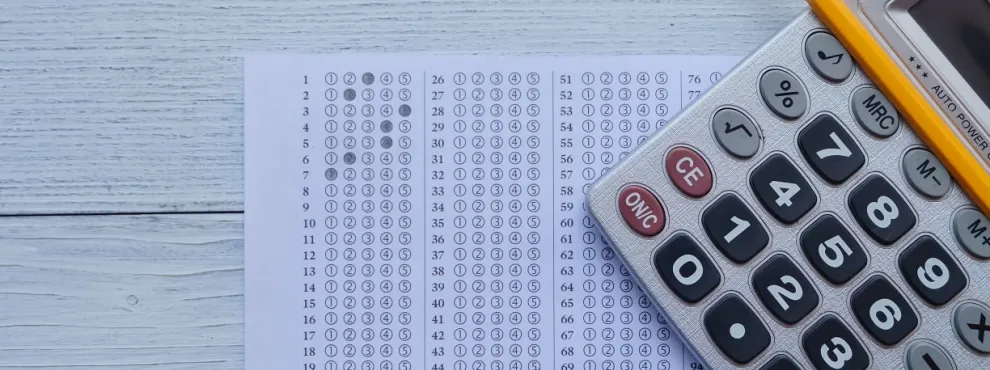Universities

# RGPV CGPA to Percentage Calculator: How to Convert CGPA to Percentage in RGPV

By Edvoy• Last updated: Oct 18, 2023Explore, filter, shortlist universities with ease and get free counselling

Achieved a CGPA of 6 or higher? Your study abroad journey starts here. Apply today!

Rajiv Gandhi Proudyogiki Vishwavidyalaya (RGPV) is a technical university in Madhya Pradesh, India. RGPV follows a 10-point CGPA (Cumulative Grade Point Average) system to grade its students. The CGPA system is a common way of evaluating a student's overall academic performance in an educational institution. However, many employers and universities prefer to assess a student's performance in the form of percentage instead of a CGPA.

If you're a student at RGPV and want to convert your CGPA to a percentage, you can follow the simple formula below.

## RGPV CGPA to Percentage Conversion Formula

To convert CGPA to percentage in RGPV, you can use the following formula:

Percentage = (CGPA/10) x 100

The formula is easy to use. All you need to know is your CGPA, which is typically mentioned on your transcript or mark sheet.

Let's take an example to understand the conversion process better. Suppose a student has a CGPA of 8.5. To convert this score to a percentage, we can use the above formula as follows:

Percentage = (8.5/10) x 100 = 85%

Therefore, the percentage equivalent of a CGPA of 8.5 in RGPV is 85%.

### How to convert CGPA into percentage in RGPV?

To calculate your CGPA into percentage, please using the following formula Percentage = (CGPA/10) x 100

### RGPV CGPA to Percentage Calculator

Achieved a CGPA of 6 or higher? Your study abroad journey starts here. Apply today!

Explore, filter, shortlist universities with ease and get free counselling

## RGPV CGPA to Percentage Conversion Certificate

Once you have calculated your percentage equivalent, you may need to obtain a certificate or document proving your percentage. RGPV provides a Conversion Certificate to students who want to convert their CGPA to percentage. The certificate includes the following details:

• Student's name
• Roll number
• CGPA obtained
• Percentage equivalent
• Date of issuance
• Signature of the authorized person

To obtain a Conversion Certificate, you can contact the Controller of Examination at RGPV or visit the official website. The certificate can be useful for further studies, job applications, or any other purpose where a percentage is required instead of a CGPA.

In summary, converting CGPA to percentage in RGPV is a simple process. You can use the formula Percentage = (CGPA/10) x 100 to calculate the percentage equivalent of your CGPA. RGPV also provides a Conversion Certificate for students who require documentation of their percentage. By following the steps mentioned in this article, you can easily convert your CGPA to a percentage and showcase your academic performance to potential employers and educational institutions.

Related pages,

## FAQ’s

### Q: What is CGPA, and how is it calculated in RGPV?

A: Cumulative Grade Point Average (CGPA) is an average of all the grade points obtained by a student in all the subjects/courses during a particular academic program. RGPV calculates CGPA on a scale of 10, where the minimum is 0, and the maximum grade point is 10.

### Q: Why do employers and universities prefer percentage over CGPA?

A: While CGPA is an effective way of evaluating a student's overall performance, employers and universities may prefer percentages for a more detailed evaluation of a student's academic performance. Percentage provides a more accurate picture of a student's grades and helps employers and universities compare the performance of students across different universities.

### Q: How do I know my CGPA in RGPV?

A: RGPV typically mentions the CGPA on the student's transcript or mark sheet. If you are not sure, you can contact the Controller of Examination at RGPV for clarification.

Explore, filter, shortlist universities with ease and get free counselling

### Q: Is it necessary to convert CGPA to percentage in RGPV?

A: No, it is not necessary to convert CGPA to percentage in RGPV. CGPA is a widely recognized grading system, and employers and universities are aware of its significance. However, if you need a percentage for any specific purpose, you can use the formula mentioned in the article to calculate the percentage equivalent to your CGPA.

### Q: How long does it take to obtain the Conversion Certificate from RGPV?

A: The time taken to obtain the Conversion Certificate from RGPV may vary depending on the university's procedures and the availability of staff. It is recommended to check with the Controller of Examination or visit the official website for updates on the same.

### Q: Can I convert my CGPA to percentage using a different formula than the one mentioned in the article?

A: No, it is not recommended to use a different formula to convert CGPA to percentage in RGPV. The formula Percentage = (CGPA/10) x 100 is a standard formula accepted by most employers and universities. Using a different formula may result in incorrect calculations and misinterpretation of grades.

Start your journey and begin a conversation with a friendly Edvoy Counsellor and download the app today.

Achieved a CGPA of 6 or higher? Your study abroad journey starts here. Apply today!

Related tags:
0 Likes